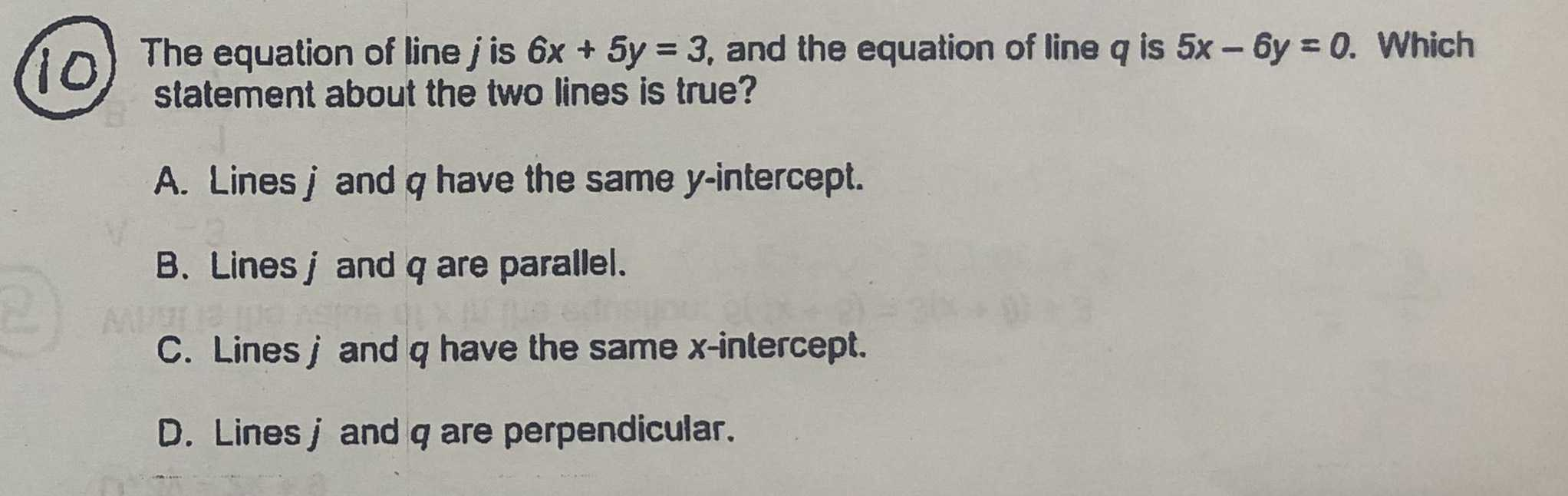### ¿Todavía tienes preguntas de matemáticas?

Pregunte a nuestros tutores expertos
Algebra
Pregunta(1) The equation of line $$j$$ is $$6 x + 5 y = 3$$ , and the equation of line $$q$$ is $$5 x - 6 y = 0$$ . Which statement about the two lines is true? A. Lines $$j$$ and $$q$$ have the same $$y$$ -intercept. B. Lines $$j$$ and $$q$$ are parallel. C. Lines $$j$$ and $$q$$ have the same $$x$$ -intercept. D. Lines $$j$$ and $$q$$ are perpendicular.